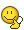BrainDen.com - Brain Teasers

Question

The first funny number: If a 1 is placed after this five-digit number, the result is triple the number you'd get by putting the 1 in front. The second funny number: It's the second-smallest odd number greater than 1 that's a perfect cube and also a perfect square. The third funny number is the smallest with the property that if any prime between 10 and 20 is divided into it, the remainder is 1. What's the exact product of the three funny numbers?

Recommended Posts

• 0

Funny Number 1: 42857

428571 * 3 = 142857

Funny Number 2: 15625

15625 = 1252 = 253

(729 = 272 = 93 is the first)

Funny Number 3: 46190

Product of Funny Numbers: 30930700468750

Edited by BobbyGo
Share on other sites

• 0

*Tried to edit original answer to fix mistake, but was too late.

Funny Number 1: 42857
142857 * 3 = 428571

Funny Number 2: 15625
15625 = 1252 = 253
(729 = 272= 93 is the first)

Funny Number 3: 46190

46190 = (11 * 13 * 17 * 19) + 1

int main()
{
int x = 20, y = 0;

for(x; y<1; x++)
{
if(x%11==1 and x%13==1 and x%17==1 and x%19==1)
{
y=1;
}
}
x=x-1;

cout<<x<<endl;
return 0;
}

Product of Funny Numbers: 30930700468750

Edited by BobbyGo
Share on other sites

• 0

 Say the first number is ‘abcde’

Then putting 1 before and after we get 1abcde * 3 = abcde1
Here 3 * e = x1, so e = 7
Then we have 1abcd7 * 3 = abcd71
Here 3 * d + carryover 2 = x7, therefore d = 5
Then we have 1abc57 * 3 = abc571
Now 3 * c + carryover 1 = x5, therefore c = 8
Then we have 1ab857 * 3 = ab8571
Now we have 3 * b + carryover 2 = x8, therefore b = 2
Then 1a2857 * 3 = a28571
Here 3 * a = x2, so a = 4,
Then finally we have 142857 * 3 = 428571
 Second smallest odd number which is perfect cube and perfect square.
Say it is a^3 which is equal to b^2. Here a & b both should be odd numbers to get required odd number.
Then a = (b/a)^2 = c^2 (say)
Then first smallest odd number which is greater than 1, and is a square of an odd number is 9, and second smallest odd number which is a square of an odd number is 25,
So 25^3 = b^2 = 125^2 = 15625

Third funny number will be worked out tomorrow...

Share on other sites

• 0

I got the same 1st and 2nd funny numbers as the previous posters, but the way I read the OP the third funny number is 2. Every prime between 10 and 20 divided by 2 will produce the remainder of 1.

So the product of the funny numbers is 1339281250.

Share on other sites

• 0

I got the same 1st and 2nd funny numbers as the previous posters, but the way I read the OP the third funny number is 2. Every prime between 10 and 20 divided by 2 will produce the remainder of 1.

So the product of the funny numbers is 1339281250.

" by " is not " into "

Share on other sites

• 0

I got the same 1st and 2nd funny numbers as the previous posters, but the way I read the OP the third funny number is 2. Every prime between 10 and 20 divided by 2 will produce the remainder of 1.

So the product of the funny numbers is 1339281250.

" by " is not " into "

It isn't indeed. And English is my second language, so all this time I've thought that it meant the same thing when applied to division.So, BobbyGo has it then...

Share on other sites

• 0

It isn't indeed. And English is my second language, so all this time I've thought that it meant the same thing when applied to division.So, BobbyGo has it then...

Unless superprismatic is right. I didn't even consider that the third number might be less than the primes instead of greater.

Join the conversation

You can post now and register later. If you have an account, sign in now to post with your account.×   Pasted as rich text.   Paste as plain text instead

Only 75 emoji are allowed.

×   Your previous content has been restored.   Clear editor

×   You cannot paste images directly. Upload or insert images from URL.